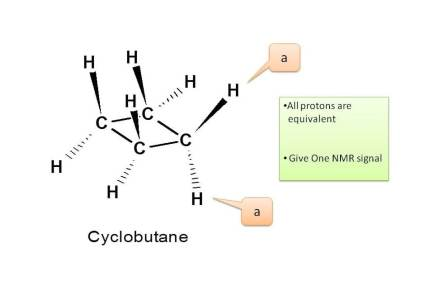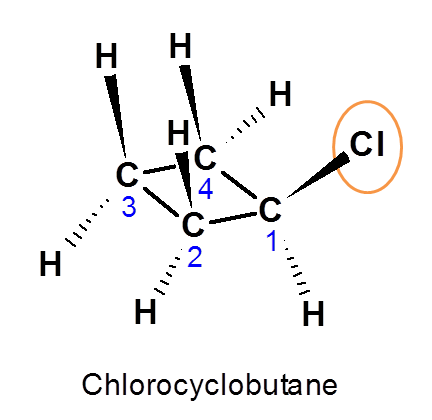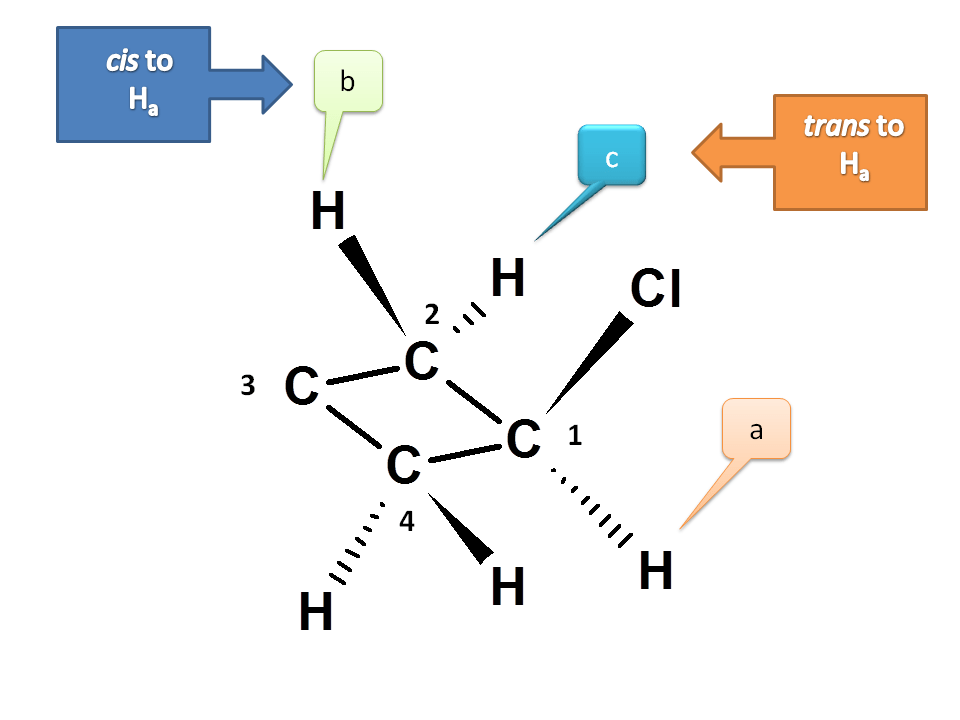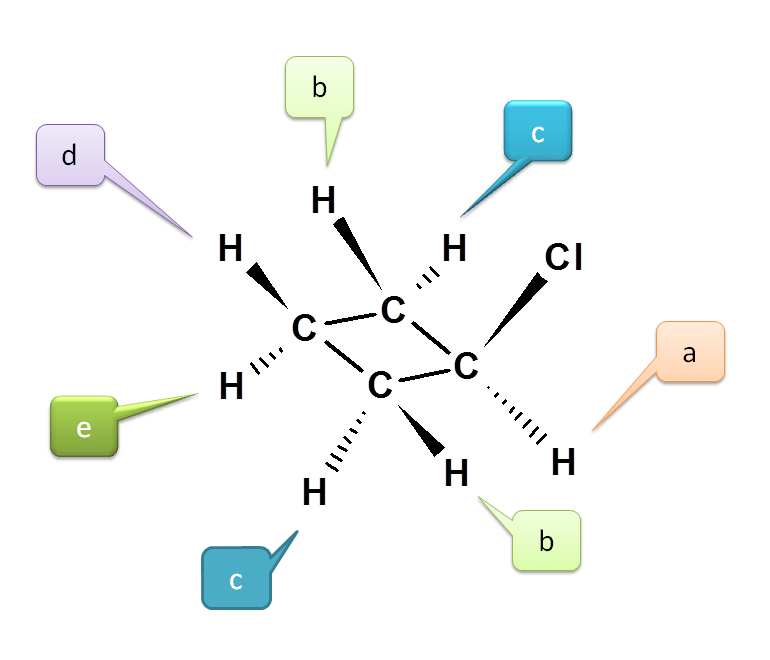1. Home
2. â€º
3. Blog
4. â€º

# Number of NMR signals in cyclic compounds

by egpat       12-06-2017

In the previous article we have already discussed how to assess the number of NMR signals in aliphatic compounds. In this article we will discuss about possible NMR signals in cyclic compounds.

For aliphatic compounds, please see How to determine number of NMR peaks.

Configuration of cyclic compounds

Cyclic compounds will have restricted rotation hence exhibit configurational isomerism. Various projection formulae can used to study the configuration of cyclic compounds on plain paper. Given below is the solid wedge-dashed line projection formula of cyclobutane.The groups projecting towards the reader are indicated by solid wedge and those groups away from the reader by dashed lines.

So here the protons indicated by solid wedge and dashed line may have either similar or different configuration depending on the substituents on cyclic compound. Let’s start with simple example.

Example 1: CyclobutaneNow in cyclobutane all carbons have same protons hence all these are equivalent. Therefore total number of NMR signals is ONE.

Example 2: Chlorocyclobutane

Let’s consider the case of chlorocyclobutane. By introduction of chlorine group now all protons are not equivalent as they have attached by different linkage.Proton attached at C1 is indicated by a (Denoted as Ha).Now let us see the protons at C2. One proton is cis and another is trans to proton at C1 i.e. Ha. Hence they are magnetically not equivalent. Therefore they are indicated by b and c.We can also observe the symmetry of C2 and C4. Hence protons at C4 are equivalent to protons at C2.Now consider C3. Again the two protons are not equivalent due to cis-trans positions with respect to chloride. Hence they are different and indicated by d and e.Hence the total number of NMR signal possible is 5.